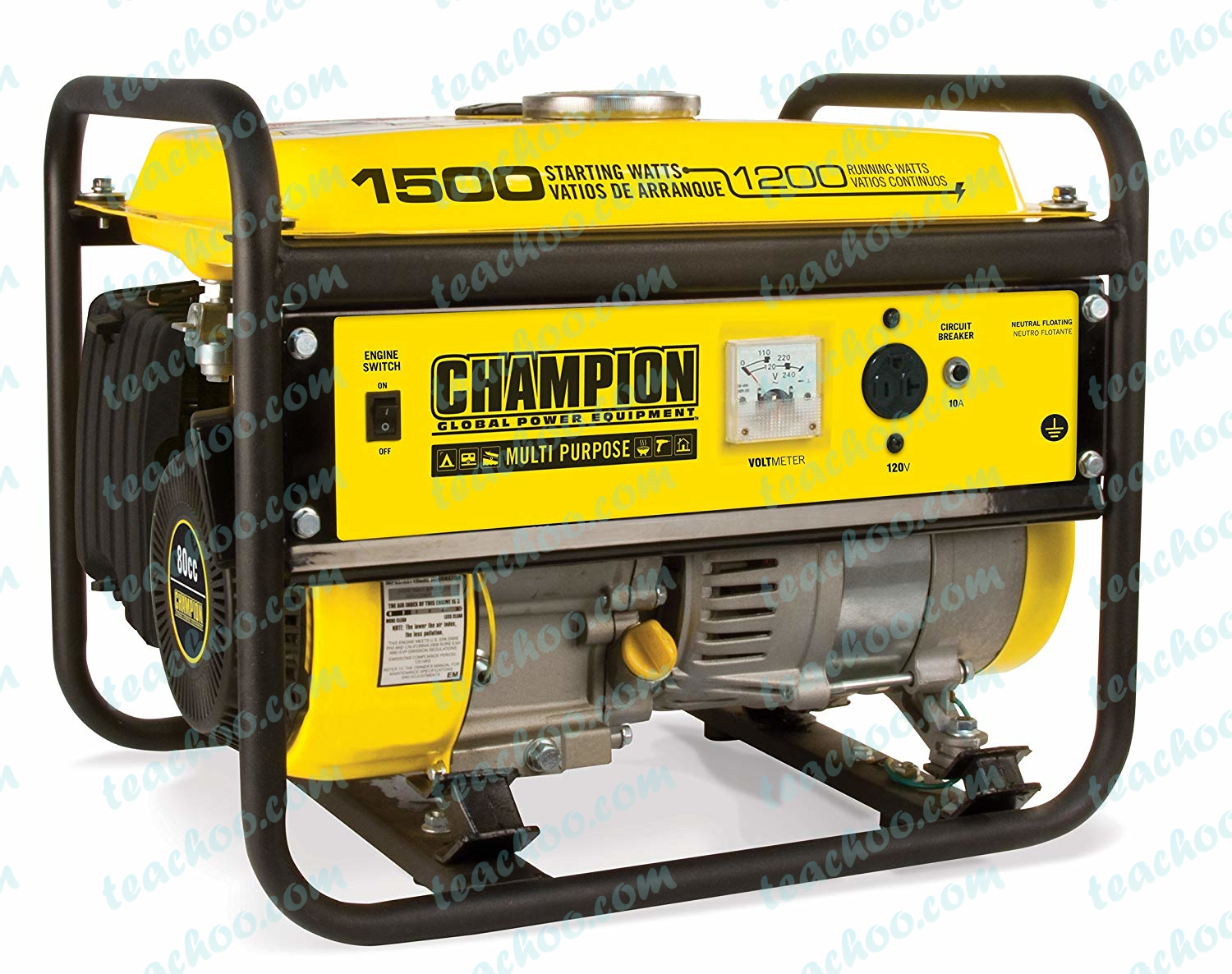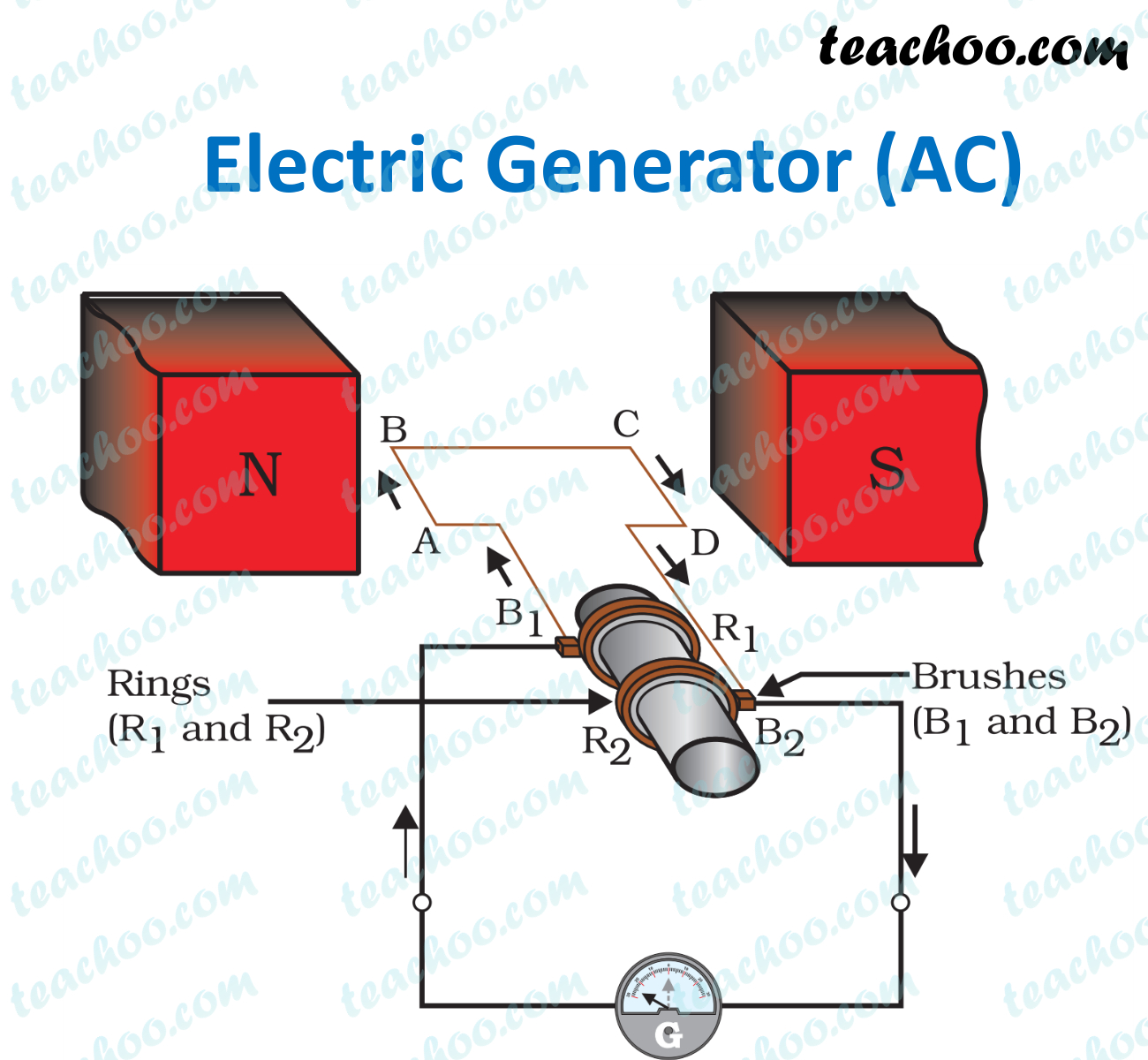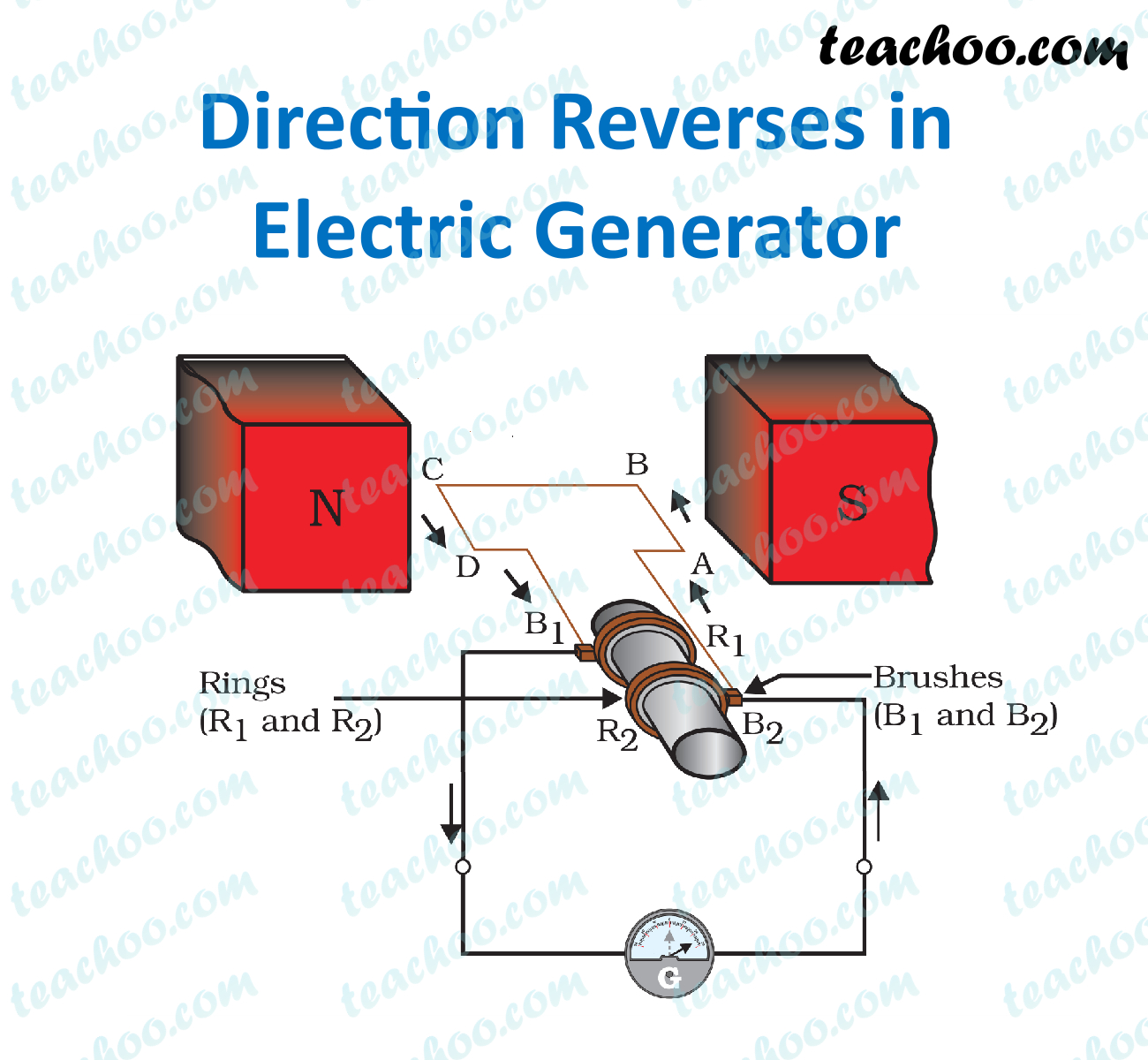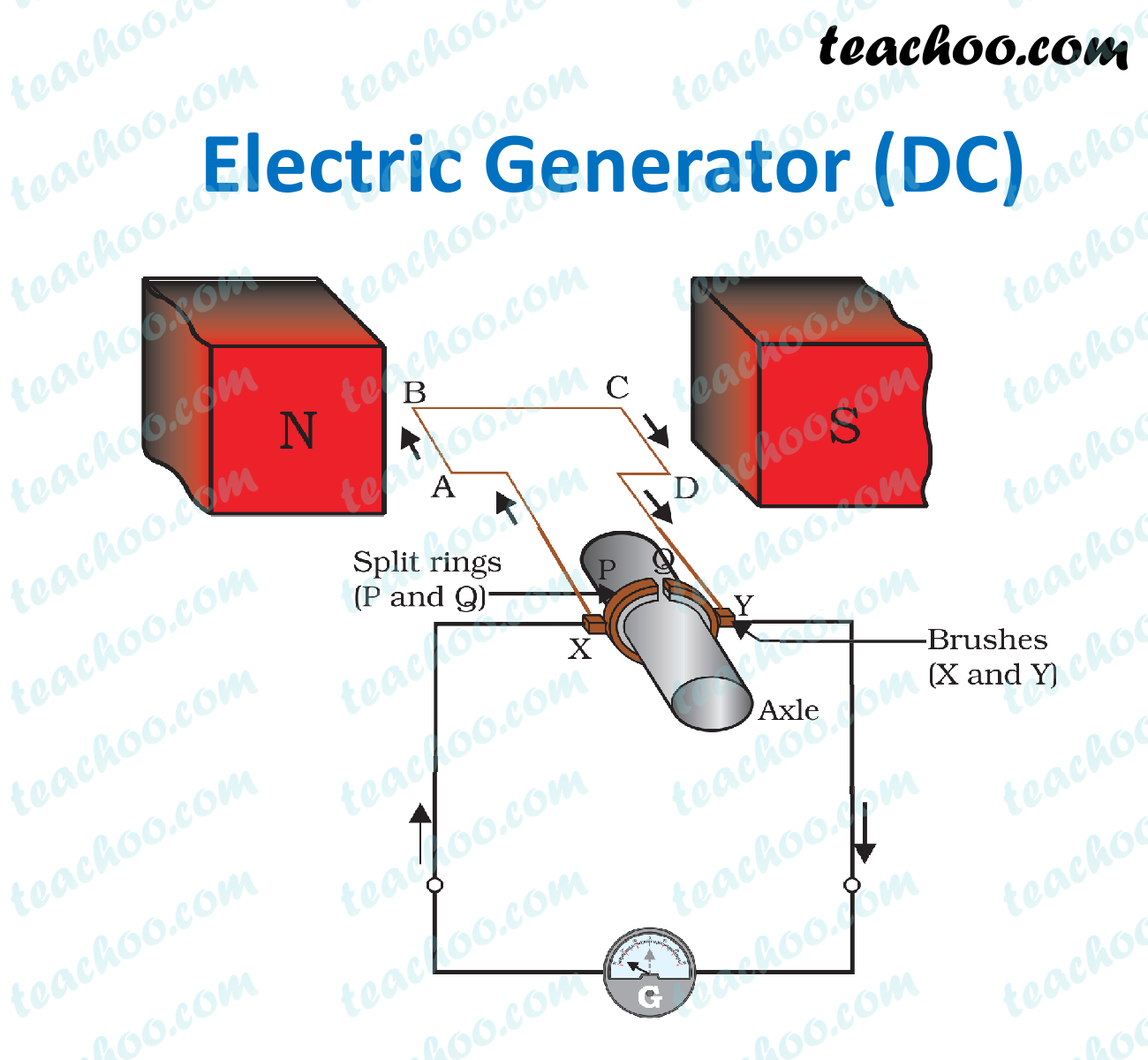Concepts

Class 10
Chapter 13 Class 10 - Magnetic Effects of Electric Current

## What is an electric generator?

An electric generator is a device which converts mechanical energy into electrical energy.

It looks like## Principle of Electrical generator

The electrical generator works on the principle that

when a straight conductor is moved in a magnetic field,

then current is induced in the conductor.

## Types of Generators

Generator is used to generate electric current.

The electric current can be - AC current or DC current.

Thus, Electric Generators is of two types

• AC Generator - Generates Alternating Current (AC current)
• DC Generator - Generates Direct Current (DC current)

Note : Whenever Electric Generator is mentioned, we will assume it is a AC Generator

## Construction of an AC Electric GeneratorAC Electric Generator consists of

• Rectangular Coil of Wire ABCD
• A strong horseshoe magnet (or 2 different magnets ) - If we take 2 magnets, North Pole of first magnet faces South Pole of Other Magnet, as shown in figure...
• The coil is placed perpendicular to the magnet as shown in figure
• The ends of coil are connected to two rings - R 1 and R 2
• The external conducting edges of rings R 1 and R 2 are connected to two stationary brushes - B 1 & B 2 respectively
• The inner side of the rings is insulated and attached to the axle
The axle is mechanically rotated to rotate the coil
• These brushes are attached to a galvanometer to show the flow of current in the circuit

## Working of an AC Electric Generator

Let's look at the working of an AC Electric Generator

• Suppose axle is rotated clockwise, so coil is also rotated clockwise,
Side AB of Coil moves up and side CD Moves down
Applying Fleming's Right Hand Rule on side AB,
force is upwards, magnetic field is left to right,
So, current flows into the paper i.e. from A to B

• And applying  Fleming's Right Hand Rule on side CD,
force is downwards, magnetic field is left to right,
So, current flows out of the paper i.e. from C to D

• Hence, current flows into the Brush B 2 , moves along galvanometer and finally enters B 1
Hence, we say current flows from B 2 to B 1 in the external circuit.

• After half a rotation,
Side CD Comes on left side and AB Comes on Right Side

• Now, on left side CD comes down,
Applying Fleming's Right Hand Rule on side CD,
force is downwards, magnetic field is left to right,
So, current flows out of the paper i.e. from D to C

• And on right side, AB comes up,
Applying Fleming's Right Hand Rule on side AB,
force is upwards, magnetic field is left to right,
So, current flows into the paper i.e. from A to B

• Hence, our circuit is now  DCBA,
and current moves in the opposite direction• Hence, we say current flows from B 1 to B 2 in the external circuit.
• Thus, after every half rotation, direction of current changes.
Hence, Alternating Current is produced

Now, lets look at DC Generator - where current is in one direction

Note: To convert AC generator to DC Generator, we use a commutator with split rings (Split, not slip). Just like we do in an Electric Motor

## Construction of DC GeneratorDC Electric Generator consists of

• Rectangular Coil of Wire ABCD
• A strong horseshoe magnet (or 2 different magnets ) - If we take 2 magnets, North Pole of first magnet faces South Pole of Other Magnet, as shown in figure...
• The coil is placed perpendicular to the magnet as shown in figure
• The ends of coil are connected to a split ring commutator - P and Q
• The external conducting edges of rings P and Q are connected to two stationary brushes - X & Y   respectively
• The inner side of the rings is insulated and attached to the axle
The axle is mechanically rotated to rotate the coil
• These brushes are attached to a galvanometer to show the flow of current in the circuit

## Working of a DC Electric Generator

Let's look at the working of a DC Electric Generator

• Suppose axle is rotated clockwise, so coil is also rotated clockwise,
Side AB of Coil moves up and side CD Moves down
Applying Fleming's Right Hand Rule on side AB,
force is upwards, magnetic field is left to right,
So, current flows into the paper i.e. from A to B

• And applying  Fleming's Right Hand Rule on side CD,
force is downwards, magnetic field is left to right,
So, current flows out of the paper i.e. from C to D

• Hence, current flows into the Brush Y , moves along galvanometer and finally enters X
Hence, we say current flows from Y to X in the external circuit.

• After half a rotation,
Side CD Comes on left side and AB Comes on Right Side

• And Split ring P is connected to coil CD and split ring Q is connected to coil AB.
Which keeps the direction of current in the circuit same.
• Hence, current flows from Brush Y , moves along galvanometer and finally enters X
Hence, we say current flows from Y to X in the external circuit.

• Thus, direction of current after every half rotation, direction of current changes.
Hence, Alternating Current is produced

## How does Power Stations increase Current and Voltage produced?

They increase current and voltage produced by

• Using Electromagnet in place of permanent magnet
• Large number of turns of conducting wire(More the turns in wire more the magnetic field)
• Soft iron Core on which coil is wound
• Rotating the coil faster

## Questions

NCERT Question 4 - The essential difference between an AC generator and a DC generator is that

1. AC generator has an electromagnet while a DC generator has permanent magnet.
2. DC generator will generate a higher voltage.
3. AC generator will generate a higher voltage.
4. AC generator has slip rings while the DC generator has a commutator.

NCERT Question 6 (b) - State whether the following statements are true or false.

An electric generator works on the principle of electromagnetic induction.

NCERT Question 16 -  The essential difference between an AC generator and a DC generator is that

Question 1 Page 237 - State the principle of an electric generator.

Question 4 Page 237 - A rectangular coil of copper wire is rotated in a magnetic field. The direction of the induced current changes once in each

(a) two revolutions (b) one revolution

(c) half revolution (d) one-fourth revolution

Learn in your speed, with individual attention - Teachoo Maths 1-on-1 Class